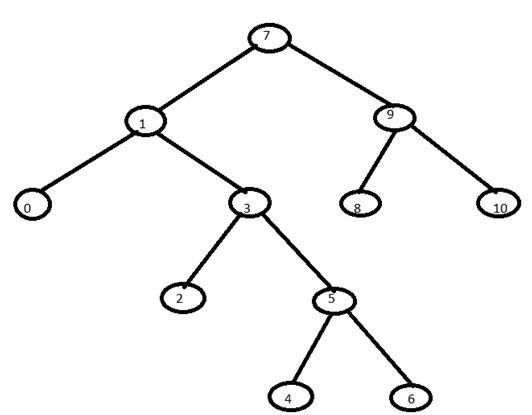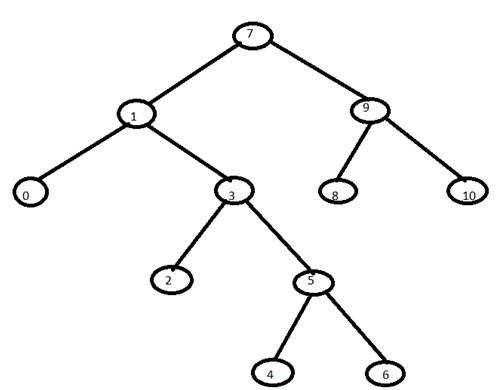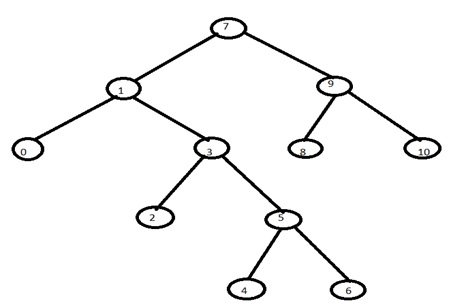# Traversal technique for Binary Tree

Learn: In this article, we will learn about Traversal technique for Binary tree with their algorithms and example.
Submitted by Abhishek Kataria, on June 11, 2018

## Binary Tree

A binary tree is a finite collection of elements or it can be said it is made up of nodes. Where each node contains the left pointer, right pointer, and a data element. The root pointer points to the topmost node in the tree. When the binary tree is not empty, so it will have a root element and the remaining elements are partitioned into two binary trees which are called the left pointer and right pointer of a tree.

## Traversing in the Binary Tree

Tree traversal is the process of visiting each node in the tree exactly once. Visiting each node in a graph should be done in a systematic manner. If search result in a visit to all the vertices, it is called a traversal. There are basically three traversal techniques for a binary tree that are,

1. Preorder traversal
2. Inorder traversal
3. Postorder traversal

### 1) Preorder traversal

To traverse a binary tree in preorder, following operations are carried out:

1. Visit the root.
2. Traverse the left sub tree of root.
3. Traverse the right sub tree of root.

Note: Preorder traversal is also known as NLR traversal.

Algorithm:

```Algorithm preorder(t)
/*t is a binary tree. Each node of t has three fields:
lchild, data, and rchild.*/
{
If t! =0 then
{
Visit(t);
Preorder(t->lchild);
Preorder(t->rchild);
}
}
```

Example: Let us consider the given binary tree,Therefore, the preorder traversal of the above tree will be: 7,1,0,3,2,5,4,6,9,8,10

### 2) Inorder traversal

To traverse a binary tree in inorder traversal, following operations are carried out:

1. Traverse the left most sub tree.
2. Visit the root.
3. Traverse the right most sub tree.

Note: Inorder traversal is also known as LNR traversal.

Algorithm:

```Algorithm inorder(t)

/*t is a binary tree. Each node of t has three fields:
lchild, data, and rchild.*/
{
If t! =0 then
{
Inorder(t->lchild);
Visit(t);
Inorder(t->rchild);
}
}
```

Example: Let us consider a given binary tree.Therefore the inorder traversal of above tree will be: 0,1,2,3,4,5,6,7,8,9,10

### 3) Postorder traversal

To traverse a binary tree in postorder traversal, following operations are carried out:

1. Traverse the left sub tree of root.
2. Traverse the right sub tree of root.
3. Visit the root.

Note: Postorder traversal is also known as LRN traversal.

Algorithm:

```Algorithm postorder(t)

/*t is a binary tree .Each node of t has three fields:
lchild, data, and rchild.*/
{
If t! =0 then
{
Postorder(t->lchild);
Postorder(t->rchild);
Visit(t);
}
}
```

Example: Let us consider a given binary tree.Therefore the postorder traversal of the above tree will be: 0,2,4,6,5,3,1,8,10,9,7

Top MCQs

Languages: » C » C++ » C++ STL » Java » Data Structure » C#.Net » Android » Kotlin » SQL
Web Technologies: » PHP » Python » JavaScript » CSS » Ajax » Node.js » Web programming/HTML
Solved programs: » C » C++ » DS » Java » C#
Aptitude que. & ans.: » C » C++ » Java » DBMS
Interview que. & ans.: » C » Embedded C » Java » SEO » HR
CS Subjects: » CS Basics » O.S. » Networks » DBMS » Embedded Systems » Cloud Computing
» Machine learning » CS Organizations » Linux » DOS
More: » Articles » Puzzles » News/Updates